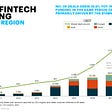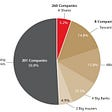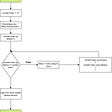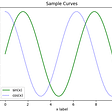# Portfolio Optimization Using Python [Part 2/2]

We will now go through another popular theory developed by William Sharpe, John Lintner, and Jan Mossin — Capital Asset Pricing Model (CAPM).

# What is CAPM?

The capital asset pricing model (CAPM) is a mathematical model that seeks to explain the relationship between risk and return in a rational equilibrium market. Developed by academia, the CAPM has been employed in applications ranging from corporate capital budgeting to setting public utility rates. The CAPM provides much of the justification for the trend toward passive investing in large index mutual funds. The Capital Asset Pricing Model (CAPM) is a model that describes the relationship between the expected return and risk of investing in a security. It shows that the expected return on a security is equal to the risk-free return plus a risk premium, which is based on the beta of that security.

The goal of the CAPM formula is to evaluate whether a stock is fairly valued when its risk and the time value of money are compared to its expected return.
Mathematically, we can define the CAPM formula as follows:

where:

• rᵢ is the expected return of a security
• r𝒻 is the risk-free rate
• βᵢ is the beta of the security relative to the market
• rₘ−r𝒻 is the risk premium

Let’s define these terms first, and then we will put the CAPM to action in Python.

## Beta

The beta (denoted as “βᵢ” in the CAPM formula) is a measure of a stock’s risk (volatility of returns) reflected by measuring the fluctuation of its price changes relative to the overall market. In other words, it is the stock’s sensitivity to market risk. For instance, if a company’s beta is equal to 1.5 the security has 150% of the volatility of the market average. However, if the beta is equal to 1, the expected return on a security is equal to the average market return. A beta of -1 means security has a perfect negative correlation with the market.

The CAPM formula is used for calculating the expected returns of an asset. It is based on the idea of systematic risk (otherwise known as non-diversifiable risk) that investors need to be compensated for in the form of a risk premium. A risk premium is a rate of return greater than the risk-free rate. When investing, investors desire a higher risk premium when taking on more risky investments. The market risk premium represents the additional return over and above the risk-free rate, which is required to compensate investors for investing in a riskier asset class. Put another way, the more volatile a market or an asset class is, the higher the market risk premium will be.

# Assumptions of CAPM

As with many financial models, not all the complexities of the financial markets are accounted for. CAPM make the following assumptions:

## Investors are risk-averse, utility-maximizing and rational individuals

This assumption does not require all investors to have the same degree of risk-aversion; it simply requires investors to be risk-averse as opposed to risk-neutral or risk-seeking. Investors are assumed to be rational if they correctly evaluate all available information to arrive at rational decisions. The rationality of investors has been called into question, as personal bias can result in irrational decision-making. However, this behavior does not affect the model outcome.

## Markets are frictionless, including no transaction costs and no taxes

In addition to assuming no transaction costs, the model also assumes investors can borrow and lend at the risk-free rate. The transaction costs of many large institutions are negligible and many investors do not pay taxes. The practical inability to borrow or lend at the risk does not materially affect the CAPM results, but costs and restrictions on short-selling can introduce an upward bias on asset prices which does affect the CAPM conclusions.

## Investors plan for the same, single holding period

The assumption of a single holding period is convenient as multi-period models become very difficult. There are shortcomings with the single-period assumption; however, it does not severely limit the applicability of the CAPM.

## Investors have homogenous expectations or beliefs

This assumption assumes all investors analyze securities, in the same way using the same probability distributions and inputs for future cash flows. This then means that all asset valuations are identical and the same optimal portfolio of risky assets is generated — the market portfolio. This assumption can be relaxed as long as the generated optimal risky portfolios are not significantly different.

## All investments are infinitely divisible

This assumes investors can hold fractions of assets and is convenient from a modelling perspective as it allows for continuous rather than discrete jump functions.

## Investors are price takers

If investors are price takers, it means no one investor can influence prices by their trades. This assumption is generally true in practice.

# Practical Implementation in Python

Let’s now dive into the practical implementation in Python:

`# Importing librariesimport numpy as npimport pandas as pdfrom pandas_datareader import data as wbimport statsmodels.api as smfrom statsmodels import regression`

We will extract daily adjusted closing values for Amazon and SPDR S&P 500 Trust ETF. The SPDR S&P 500 trust is an exchange-traded fund which trades on the NYSE Arca under the symbol. SPDR is an acronym for the Standard & Poor’s Depositary Receipts, the former name of the ETF. It is designed to track the S&P 500 stock market index. This fund is the largest ETF in the world. We will extract the data from 2008–01–01 till 2021–02–14.

`# Creating a list of Stock Tickersstocks = ['AMZN','SPY']pf_data = pd.DataFrame()# Pulling closing price   for stock in stocks:    pf_data[stock] = wb.DataReader(stock, data_source = 'yahoo', start = '2008-1-1')['Adj Close']num_stocks = len(stocks)`

Next, we will compute the daily log returns.

`# Compute log returnssec_returns = np.log(pf_data / pf_data.shift(1))`

Now, we will calculate the covariance matrix and extract the covariance value of Amazon and S&P 500 index. As earlier, we will use 252 days to annualize the measures.

`cov = sec_returns.cov() * 252cov_with_market = cov.iloc[0,1]`

We also need the market variance to calculate the Beta.

`# Calculating BetaAMZN_beta = cov_with_market / market_varround(AMZN_beta,2)>>> 1.04`

The beta value shows that Amazon is 4% more volatile than the S&P 500.

Finally, we compute the expected return of Amazon as per the CAPM. We will take the risk-free return as 1.21% which was the closing rate of 10-year US Government Bonds on 2021–02–12.

`# Calculate the expected returnAMZN_er = 0.0121 + AMZN_beta * 0.05round(AMZN_er,2)>>> 0.06`

The Amazon stock has an expected return rate of 6%.

Let’s use the concept of CAPM to calculate the expected return for a portfolio of stocks. The first step is to create two empty dictionaries that will hold the beta and alpha of each stock. Alpha describes the strategy’s ability to beat the market (S&P500):

`beta = {}alpha = {}`

Alpha is a measure of the performance of an investment as compared to a suitable benchmark index, such as the S&P 500. An alpha of one (the baseline value is zero) shows that the return on the investment during a specified time frame outperformed the overall market average by 1%. A negative alpha number reflects an investment that is underperforming as compared to the market average.

Note, alpha is a historical number. It’s useful to track a stock’s alpha over time to see how it did, but it can’t tell you how it will do tomorrow.

Let’s use the same stocks from our previous article for our portfolio. We will extract data from 2015–01–01 to date.

`# Creating a list of Stock Tickersstocks = ['HSBC','JPM','TSLA','WMT','AMZN','COST','SPY']pf_data = pd.DataFrame()# Pulling closing price   for stock in stocks:    pf_data[stock] = wb.DataReader(stock, data_source = 'yahoo', start = '2015-1-1')['Adj Close']num_stocks = len(stocks)`

We need the daily percentage change for these securities.

`# Daily percentage changepf_data_returns = pf_data.pct_change(1)pf_data_returns = pf_data_returns[1:]`

The next step is to create two empty dictionaries that will hold the beta and alpha of each stock. Alpha describes the strategy’s ability to beat the market (S&P500):

`beta = {}alpha = {}`

Next, we will loop through the daily returns of each stock and perform the following steps:

• We need to ignore the Date and S&P 500 columns.
• Plot a scatter plot of each daily stock return and the daily market return.
• Fit a polynomial line between each stock and the market and plot the best fit line.
• Update the beta and alpha dictionaries.
`for i in pf_data_returns.columns:    if i != 'SPY':        pf_data_returns.plot(kind = 'scatter', x = 'SPY', y = i)        b,a = np.polyfit(pf_data_returns['SPY'], pf_data_returns[i],    1)        plt.plot(pf_data_returns['SPY'], b * pf_data_returns['SPY'] + a, '-', color = 'r')          beta[i] = b            alpha[i] = a        plt.show()`

The beta and alpha dictionaries contain the beta and alpha values for each stock. Now that we have the beta of each stock we can calculate the CAPM. First, we will obtain a list with all the stock names called `keys`:

`keys = list(beta.keys())`

Next, we will define an empty dictionary for the expected returns of each stock. We’ll also set the risk-free rate to 0 and get the annual return of the market:

`ER = {}rf = 0 rm = pf_data_returns['SPY'].mean() * 252`

Next, we will create a `for` loop and apply the CAPM formula to each stock as follows:

`for i in keys:    ER[i] = rf + (beta[i] * (rm-rf))    print('Expected Return based on CAPM for {} is {}%'.format(i,round(ER[i]*100,2)))>>> Expected Return based on CAPM for HSBC is 11.5%>>> Expected Return based on CAPM for JPM is 17.77%>>> Expected Return based on CAPM for TSLA is 18.26%>>> Expected Return based on CAPM for WMT is 8.04%>>> Expected Return based on CAPM for AMZN is 13.92%>>> Expected Return based on CAPM for COST is 9.46%`

Next, we will assign equal weights to each stock in the portfolio. We can then calculate the expected portfolio return by multiplying the `portfolio_weights` by the sum of expected returns for the individual stocks:

`portfolio_weights = 1/6 * np.ones(6) ER_portfolio = sum(list(ER.values()) * portfolio_weights)print('Expected Return based on CAPM for the portfolio is {}%\n'.format(round(ER_portfolio*100,2)))>>> Expected Return based on CAPM for the portfolio is 13.16%`

The CAPM gives an expected return of 13.16% for the portfolio assuming equal weights of the securities.

# Extensions of the CAPM

In 1976, S. Ross published a different model, the Arbitrage Pricing Theory (APT), which avoids the need for specifying a market portfolio. Although this model is generally believed to be more powerful than the CAPM, it is less intuitive and more difficult to implement. In the APT, an asset’s return is related to multiple economic factors instead of the market portfolio.

Another attempt to modify the CAPM involves adjusting it for temporality. With temporal modelling, investors consider the consequences of decisions over multiple periods, instead of over the next period only, as the CAPM assumes. The resulting Consumption Capital Asset Pricing Model (CCAPM) is much more complex than its non-temporal counterpart. It has been shown to work successfully in situations where the normal CAPM has failed, most notably for forward exchange rates and for futures markets. Many other adaptations of the CAPM are in use. It is a validation of the widespread applicability of the CAPM that so many individuals have improved, transformed, or modified it to fit specific situations.

--

--

--

## More from Aman Gupta

Is a pantomath and a former entrepreneur. Currently, he is in a harmonious and a symbiotic relationship with Data.

Love podcasts or audiobooks? Learn on the go with our new app.

## Why The Pandemic Isn’t Affecting Me Financially## Fintech: Don’t Burst the Bubble## What Are The Conventional Loan Home Condition Requirements?## 12 Serious Crafty Tips for Saving Money at Michaels## The Case for China & Emerging Market Exposure## 3 Essential Tips for Traders on How to Invest## Making Your Retirement Income Plan Tax-Efficient## Aman Gupta

Is a pantomath and a former entrepreneur. Currently, he is in a harmonious and a symbiotic relationship with Data.

## Getting Started With Python — Part 5## Mastering Python Fundamental in 3 Days | Day 3 | Looping With Python## [TUTORIAL] A BASIC DATA ANALYSIS WITH POSITIVES COVID 19 PERU USING PYTHON## Getting Started With Matplotlib Using Python